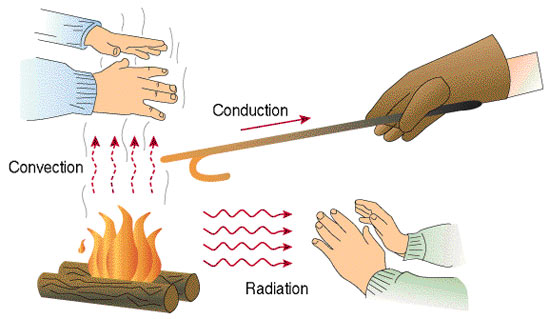# Difference Between Convection and Conduction

## Main Difference – Convection vs. Conduction

Convection and conduction are both mechanisms of heat transfer. The main difference between convection and conduction is that, in convection, heat is transferred through a mass flow of material while, in conduction, heat is transferred through collisions of particles that make up the material.

## What is Conduction

Particles that make up matter are always in motion. When the temperature increases, the particles have larger kinetic energies, and consequently they vibrate with larger amplitudes. During conduction, a vibrating particle knocks a neighbouring particle, imparting energy onto that particle. This particle now vibrates with a larger amplitude, and it can collide with another neighbouring atom, giving it energy. This energy-transfer process can continue from one end of an object to the other end. Since an increase in kinetic energy of particles physically manifests as an increase in temperature, the gradual increase in the kinetic energies of particles along the object is accompanied by a gradual increase in temperature along the object. This process, whereby heat is transferred as a result of colliding particles is called conduction.

The ability of a material to transfer heat via conduction is characterised by its conductivity. The rate of heat transfer,$\frac{\mathrm dQ}{\mathrm dt}$ or the heat current, between two objects having a temperature difference of$\Delta T$ is given by$\frac{\mathrm dQ}{\mathrm dt}=-\kappa\frac{A\Delta T}{l}$

where$A$ and$l$ are the cross-sectional area and the length of the conductor transferring heat respectively. The letter$\kappa$ is the thermal conductivity, measured in units of W m-1 K-1.

As seen from the equation, the rate of heat transfer is directly proportional to the temperature difference and the cross-sectional area of the conductor, and inversely proportional to the length of the conductor. The value of thermal conductivity depends on the microscopic properties of the material. Metals are good thermal conductors because they contain a large number of free electrons that can collide freely to transfer energy. In the meantime, the ions that form the lattice vibrate about fixed positions also collide and transfer heat. However, the free electrons are responsible for most of the heat transfer in metals.

## What is Convection

Convection is the mechanism of heat transfer in materials through a mass flow of the material. Here, in order to transfer heat, parts of the material itself moves — i.e. there is a transfer of mass within the material. Typically, convection occurs in fluids. However, effects of convection can be seen sometimes in solids, as in the case of plate tectonics. The diagram below shows the swirling patterns of convection formed by steam rising from a cup of coffee:Convection currents starting to form in steam rising from a cup of hot liquid

Convection is a complex process and there is no simple equation that fully describes it. However, we can make use of an approximation for cases where a fluid is heated using a solid surface. For these cases, the heat transfer rate$\frac{\mathrm{d}Q}{\mathrm{d}t}$ is given by,$\frac{\mathrm{d}Q}{\mathrm{d}t}=hA\left( T_s-T_a\right)$

where$A$ is the surface area that heat is transferred through,$T_s$ is the temperature of the solid,$T_a$ is the temperature of air.$h$ is known as the convective heat transfer coefficient. This coefficient depends on a number of properties including the density, viscosity, and the flow rate of the fluid. The unit for convective heat transfer coefficient is W m-2 K-1.

Note that the fluids transferring heat via convection are also transferring heat via conduction. If the conduction is very effective, it can prevent the formation of convectional currents and impede the convective heat transfer. Whether the dominant heat transfer mechanism is conduction or convection for a fluid could be found by calculating a number known as the Rayleigh number.

The diagram below illustrates cases where each of the three types of heat transfer mechanism is dominant.Difference between the three main heat transfer mechanisms illustrated: the difference between convection and radiation was covered in another article.

## Difference Between Convection and Conduction

### Mechanism

Conduction transfers heat via the transfer of kinetic energy during collisions between vibrating particles.

Convection transfers heat by moving the particles that make up the material.

### Material

Conduction is typically the dominant mechanism of heat transfer in solids.

Convection is typically the dominant mechanism of heat transfer in fluids.

Image Courtesy

“Convection” by Rebecca Siegel (Own work) [CC BY 2.0], via flickr

“ไทย: http://www.roasterproject.com/2010/01/heat-transfer-the-basics/” by Kmecfiunit (Own work) [CC BY-SA 3.0], via Wikimedia Commons